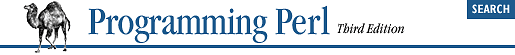home | O'Reilly's CD bookshelfs | FreeBSD | Linux | Cisco | Cisco Exam## 3.17. Assignment Operators

Perl recognizes the C assignment operators, as well as providing some of its own. There are quite a few of them:

```=    **=    +=    *=    &=    <<=    &&=
-=    /=    |=    >>=    ||=
.=    %=    ^=
x=```
Each operator requires a target lvalue (typically a variable or array element) on the left side and an expression on the right side. For the simple assignment operator:
```TARGET = EXPR
```
the value of the EXPR is stored into the variable or location designated by TARGET. For the other operators, Perl evaluates the expression:
```TARGET OP= EXPR
```
as if it were written:
```TARGET = TARGET OP EXPR
```
That's a handy mental rule, but it's misleading in two ways. First, assignment operators always parse at the precedence level of ordinary assignment, regardless of the precedence that OP would have by itself. Second, TARGET is evaluated only once. Usually that doesn't matter unless there are side effects, such as an autoincrement:
```\$var[\$a++] += \$value;               # \$a is incremented once
\$var[\$a++] = \$var[\$a++] + \$value;   # \$a is incremented twice```
Unlike in C, the assignment operator produces a valid lvalue. Modifying an assignment is equivalent to doing the assignment and then modifying the variable to which it was assigned. This is useful for modifying a copy of something, like this:
`(\$tmp = \$global) += \$constant;`
which is the equivalent of:
`\$tmp = \$global + \$constant;`
Likewise:
`(\$a += 2) *= 3;`
is equivalent to:
```\$a += 2;
\$a *= 3;```
That's not terribly useful, but here's an idiom you see frequently:
`(\$new = \$old) =~ s/foo/bar/g;`
In all cases, the value of the assignment is the new value of the variable. Since assignment operators associate right-to-left, this can be used to assign many variables the same value, as in:
`\$a = \$b = \$c = 0;`
which assigns 0 to \$c, and the result of that (still 0) to \$b, and the result of that (still0) to \$a.

List assignment may be done only with the plain assignment operator, =. In list context, list assignment returns the list of new values just as scalar assignment does. In scalar context, list assignment returns the number of values that were available on the right side of the assignment, as mentioned in Chapter 2, "Bits and Pieces". This makes it useful for testing functions that return a null list when unsuccessful (or no longer successful), as in:

```while ((\$key, \$value) = each %gloss) { ... }

next unless (\$dev, \$ino, \$mode) = stat \$file;```